Question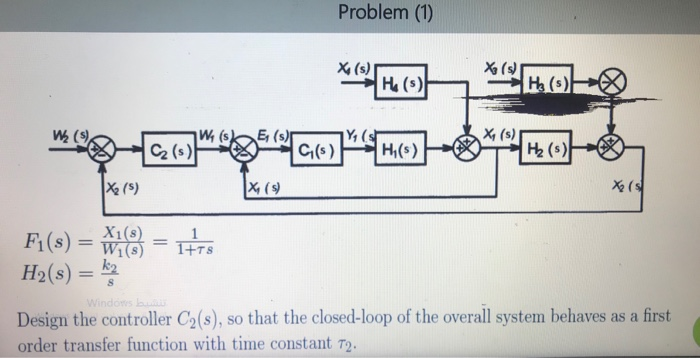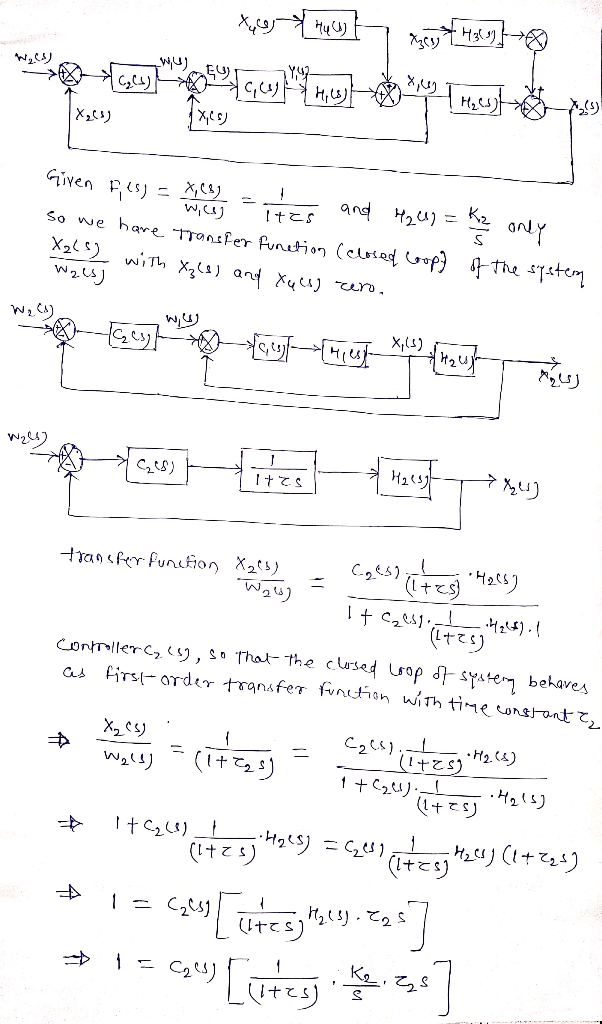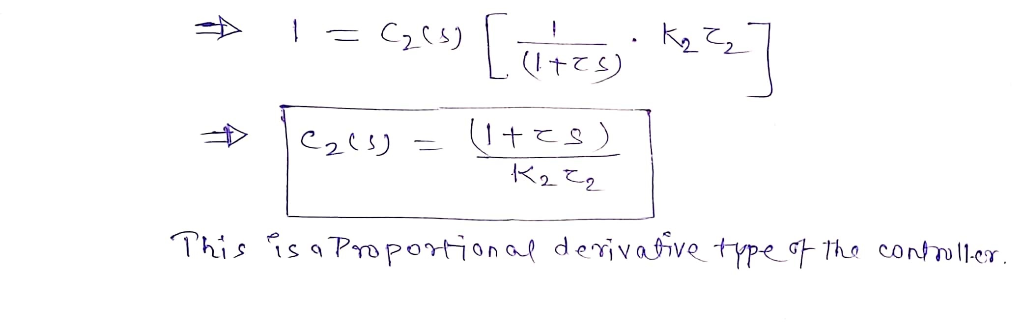#### Earn Coins

Coins can be redeemed for fabulous gifts.

Similar Homework Help Questions
• ### (s + 1) X X H(s) Figure 2 a) Find H(s) so that the closed loop...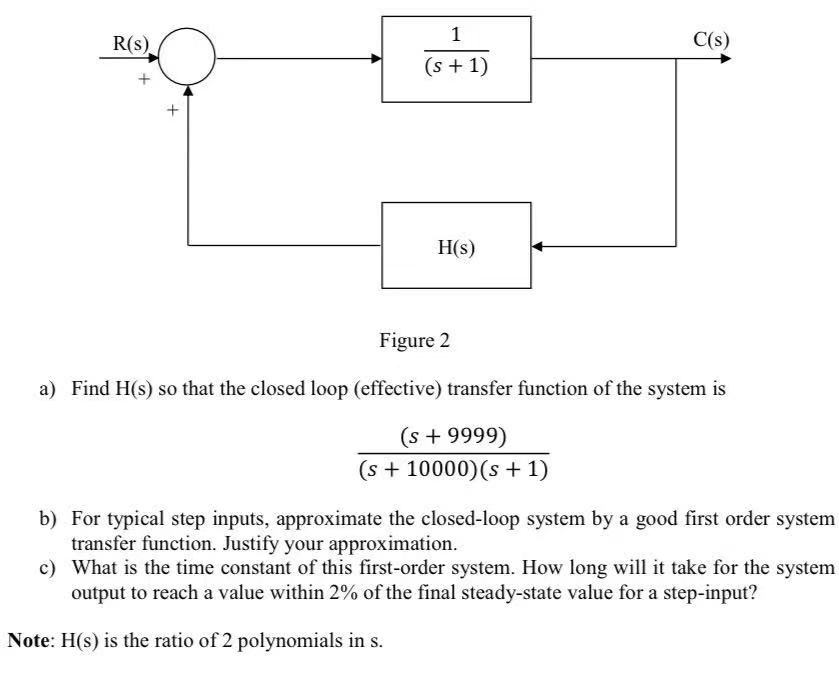(s + 1) X X H(s) Figure 2 a) Find H(s) so that the closed loop (effective) transfer function of the system is (s + 9999) (s + 10000)(8 + 1) b) For typical step inputs, approximate the closed-loop system by a good first order system transfer function. Justify your approximation. c) What is the time constant of this first-order system. How long will it take for the system output to reach a value within 2% of the final steady-state...

• ### on Matlab please!!!! Problem 1- (a) Design a controller for a plant with transfer function, G(s)-(+ to obtain (i) estep(00)s 0, (ii) T12%) < 1 s, and (iii) an-5 rad/s (4 points). (b) Plot the u...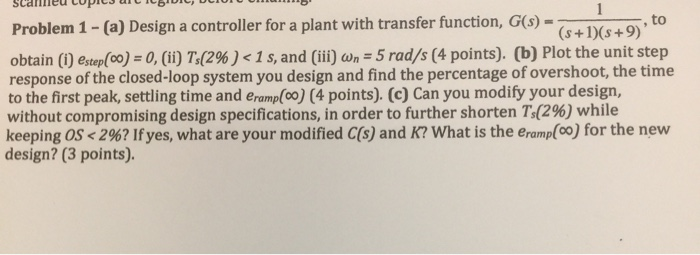on Matlab please!!!! Problem 1- (a) Design a controller for a plant with transfer function, G(s)-(+ to obtain (i) estep(00)s 0, (ii) T12%) < 1 s, and (iii) an-5 rad/s (4 points). (b) Plot the unit step response of the closed-loop system you design and find the percentage of overshoot, the time to the first peak, settling time and eramp[oo) (4 points). (c) Can you modify your design, without compromising design specifications, in order to further shorten T1296) while keeping...

• ### PD & PID controller design Consider a unity feedback system with open loop transfer function, G(s)...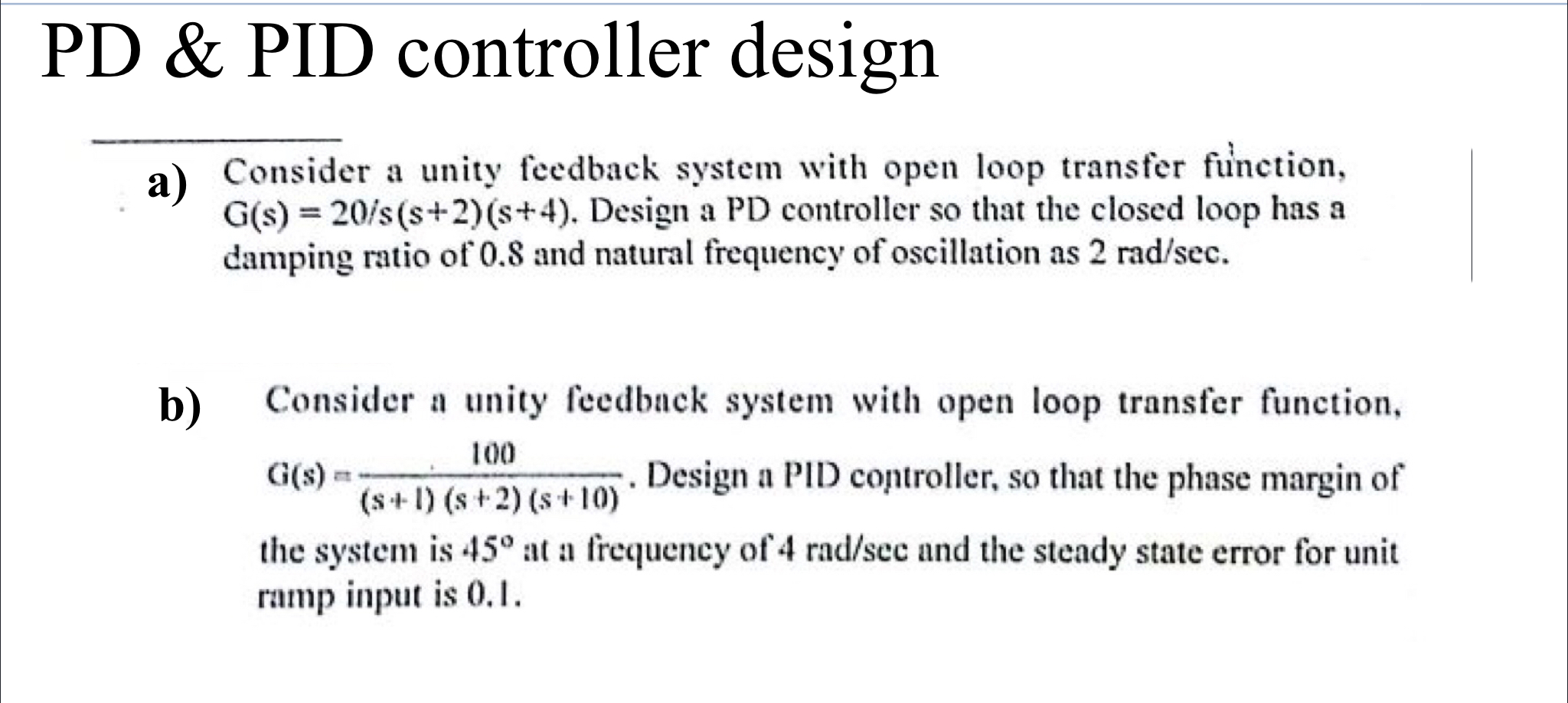PD & PID controller design Consider a unity feedback system with open loop transfer function, G(s) = 20/s(s+2)(8+4). Design a PD controller so that the closed loop has a damping ratio of 0.8 and natural frequency of oscillation as 2 rad/sec. b) 100 Consider a unity feedback system with open loop transfer function, aus. Design a PID controller, so that the phase margin of (S-1) (s + 2) (s+10) the system is 45° at a frequency of 4 rad/scc and...

• ### y(s) 2 u(s) s1 -. Consider the open-loop unstable system G(s) integral controller to regulate the output y to a constant reference r. The desired closed-loop transfer function is G) +16s +100 Design...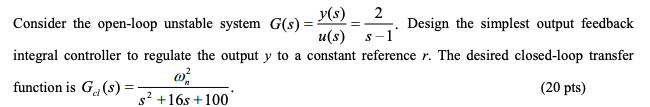y(s) 2 u(s) s1 -. Consider the open-loop unstable system G(s) integral controller to regulate the output y to a constant reference r. The desired closed-loop transfer function is G) +16s +100 Design the simplest output feedback (20 pts) y(s) 2 u(s) s1 -. Consider the open-loop unstable system G(s) integral controller to regulate the output y to a constant reference r. The desired closed-loop transfer function is G) +16s +100 Design the simplest output feedback (20 pts)

• ### 4.35 Consider the feedback control system with the plant transfer function G(s) = (5+0.1)(5+0.5) (a) Design...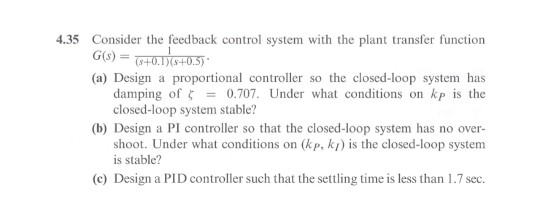4.35 Consider the feedback control system with the plant transfer function G(s) = (5+0.1)(5+0.5) (a) Design a proportional controller so the closed-loop system has damping of 5 = 0.707. Under what conditions on kp is the closed-loop system stable? (b) Design a PI controller so that the closed-loop system has no over- shoot. Under what conditions on (kp, kt) is the closed-loop system is stable? (©) Design a PID controller such that the settling time is less than 1.7 sec.

• ### Consider a unity-feedback control system with a PI controller Gpr(s) and a plant G(s) in cascade. In particular, the plant transfer function is given as 2. G(s) = s+4, and the PI controller trans...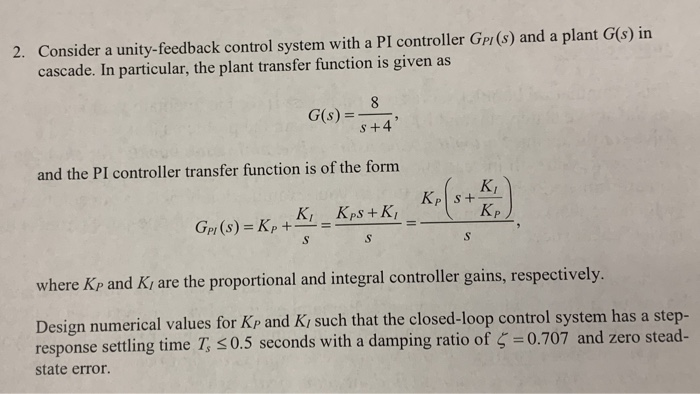Consider a unity-feedback control system with a PI controller Gpr(s) and a plant G(s) in cascade. In particular, the plant transfer function is given as 2. G(s) = s+4, and the PI controller transfer function is of the forrm KI p and Ki are the proportional and integral controller gains, respectively where K Design numerical values for Kp and Ki such that the closed-loop control system has a step- response settling time T, 0.5 seconds with a damping ratio of...

• ### Problem (2) The open loop transfer function of a feedback system is given by к H...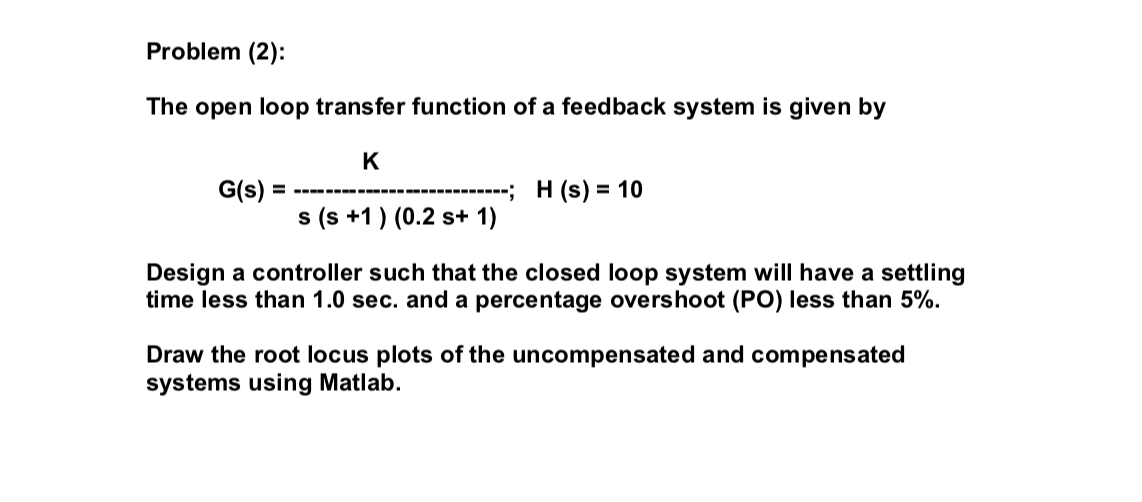Problem (2) The open loop transfer function of a feedback system is given by к H (s) = 10 G(s) = ------ - s (s +1) (0.2 s+ 1) Design a controller such that the closed loop system will have a settling time less than 1.0 sec. and a percentage overshoot (PO) less than 5%. Draw the root locus plots of the uncompensated and compensated systems using Matlab.

• ### Design a PD controller for mass-spring systems by the Root-Locus Method Mass 2.6Kg Spring stiffness 200N/m Zero Damp...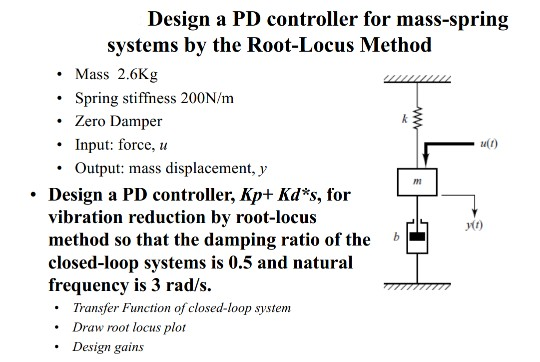Design a PD controller for mass-spring systems by the Root-Locus Method Mass 2.6Kg Spring stiffness 200N/m Zero Damper Input: force Output: mass displacement, y Design a PD controller, Kp+ Kd*s, for vibration reduction by root-locus method so that the damping ratio of the closed-loop systems is 0.5 and natural frequency is 3 rad/s Transfer Function of closed-loop system Draw root locus plot Design gains ww Design a PD controller for mass-spring systems by the Root-Locus Method Mass 2.6Kg Spring stiffness...

• ### Give me the explanation plz 2. a) A digital controller implementation for a feedback system is shown in Figure 2 where the sampling period is T0.1 second. The plant transfer function is s +10 P(s) =...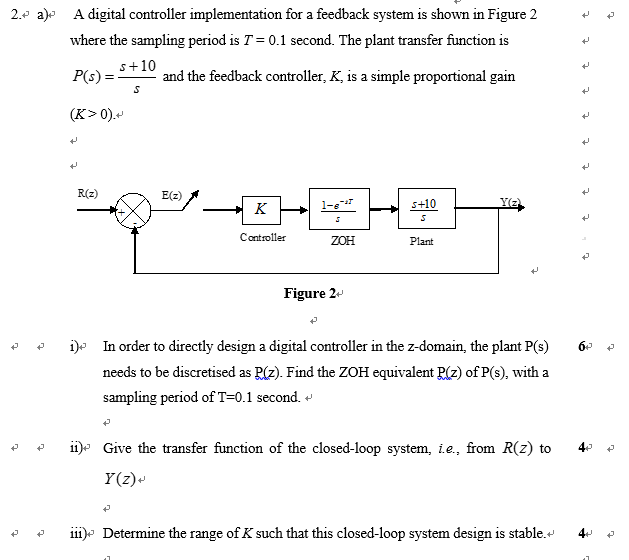Give me the explanation plz 2. a) A digital controller implementation for a feedback system is shown in Figure 2 where the sampling period is T0.1 second. The plant transfer function is s +10 P(s) = and the feedback controller, K, is a simple proportional gain (K>0).v R(z) E(z) S+10 Controller ZOH Plant Figure 2* i)o In order to directly design a digital controller in the z-domain, the plant P(s) 6. needs to be discretised as P(z). Find the ZOH...

• ### Design Problems: (1) A robotic system is described by the transfer function P(s)=- 100 s(s +9.7)(s...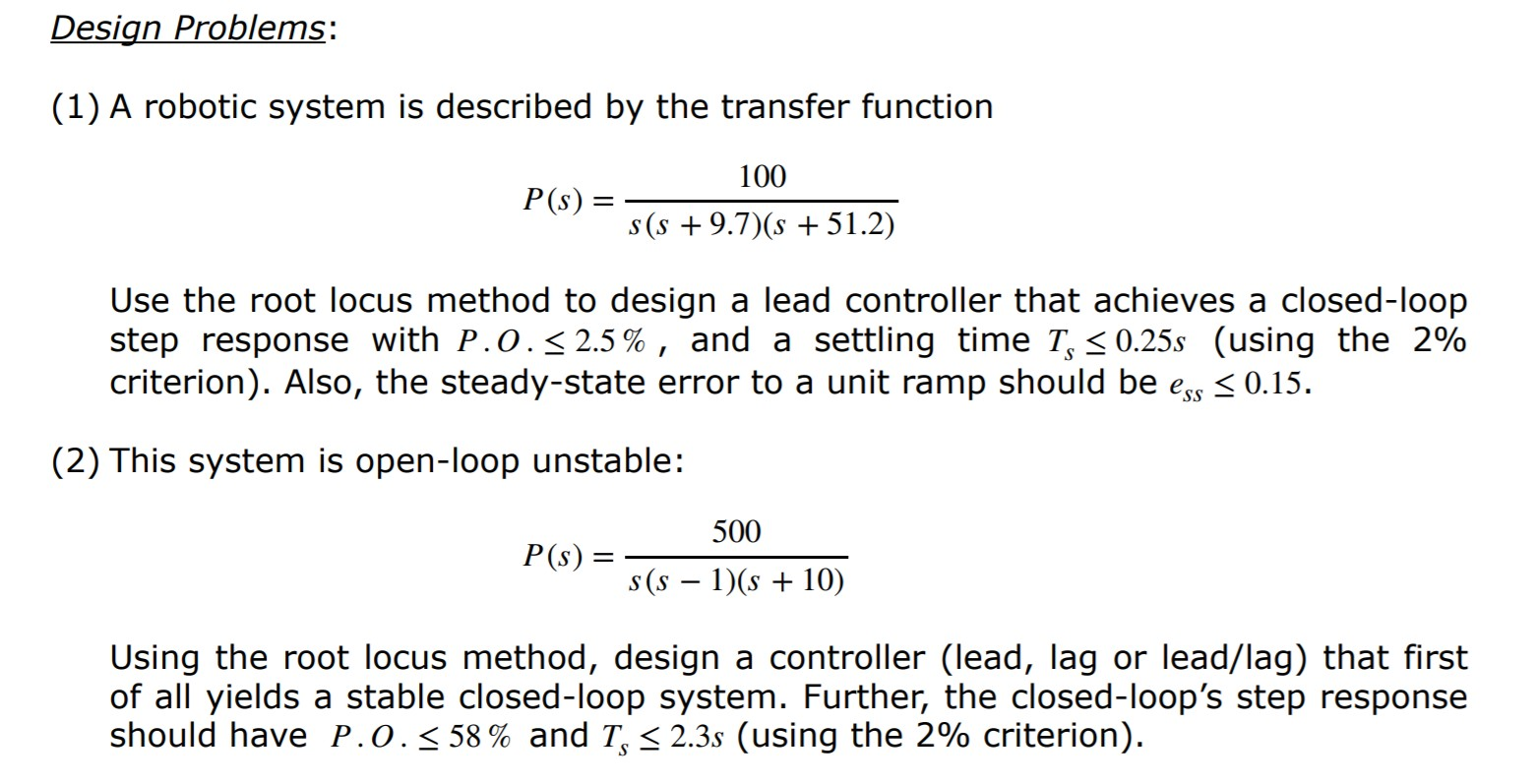Design Problems: (1) A robotic system is described by the transfer function P(s)=- 100 s(s +9.7)(s + 51.2) Use the root locus method to design a lead controller that achieves a closed-loop step response with P.0.5 2.5 %, and a settling time T, < 0.25s (using the 2% criterion). Also, the steady-state error to a unit ramp should be ess < 0.15. (2) This system is open-loop unstable: P(S) = 500 (5 - 1)(s + 10) Using the root locus...